# How to highlight only text containing cells

In this article we will learn how to apply conditional formatting in cell.

To apply Conditional formatting based on text, we use the Conditional Formatting option in Microsoft Excel.

Conditional Formatting: - It is used to highlight the important points with color in a report.

Let’s take an example and understand how we can apply formatting only to text containing cells.

We have data in range A1:C10,in which Text and numbers are miscellaneous, from that we want to highlight the cells which is contain text.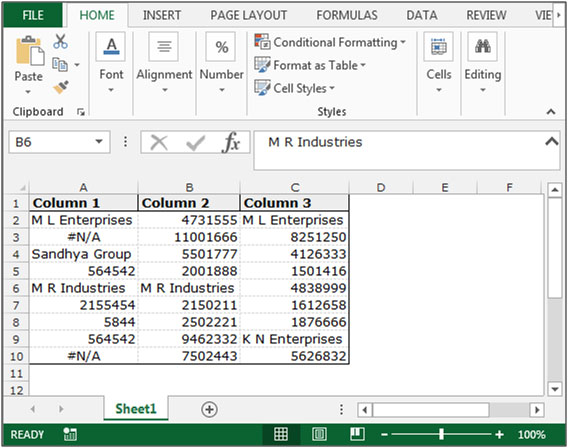Follow below given steps to highlight the text contain cells:-

• Select the data A2:C10 and Go to “Home” tab in menu bar select “Conditional Formatting” in the “Styles” group.
• Click on “Conditional Formatting” and select “New Rule” from the drop down list.• “New Formatting Rule” dialog box will appear, select a rule type “Use a formula to determine which cells to format”.
• Write the formula in the box of “Format values where this formula is true:”
• =ISTEXT(A2).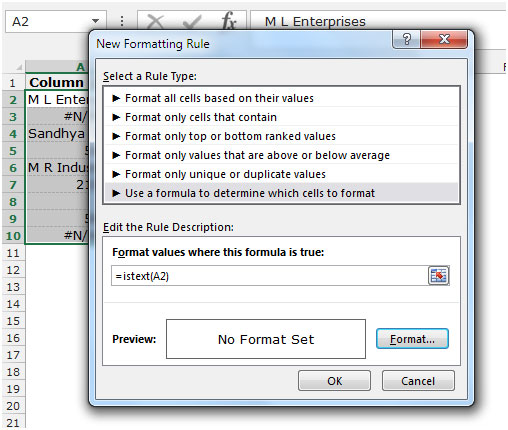• Click on “Format” button, Format Cells dialog box will appear.
• Go to Fill tab and choose the color as per the requirement and click on OK.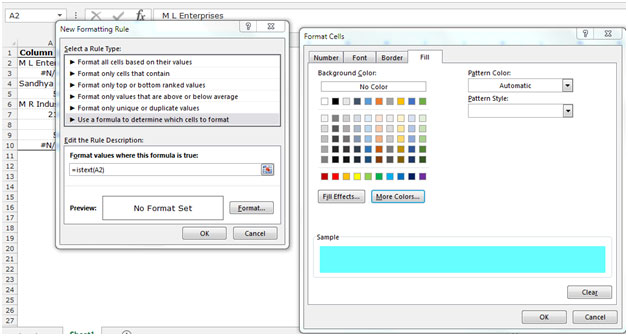• To close the “Conditional formatting” dialog box. Applying formatting only to cells contains text.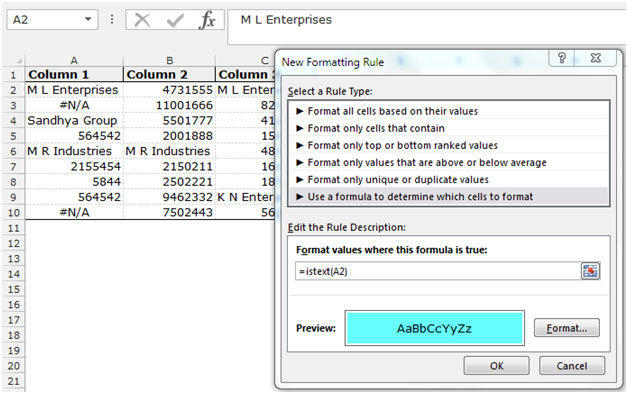• After putting the conditional formatting for text containing cells, all the text containing cells will get highlighted with the chosen color.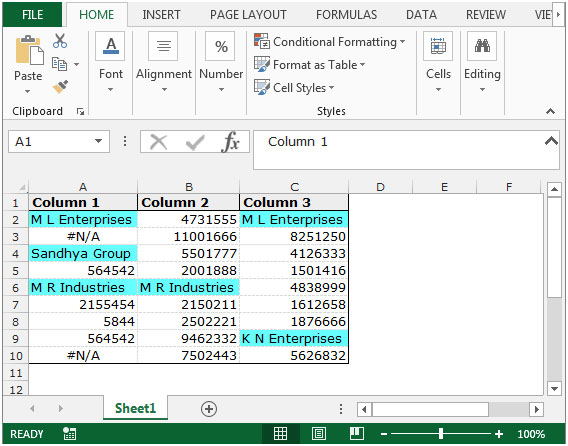This is the way you can use conditional formatting for text containing cells in Microsoft Excel 2010 and 2013.

## Users are saying about us...

1.Hi,
I am looking for the rule of conditional formatting for text where are two words in the cell.
For example, If I look for one word the rule will be ( =ISTEXT(A1) ), But if I look for two words What will be the rule?
Thanks

•If you want to highlight cells that have two words then use this formula in conditional formatting.
=COUNTIF(A2," ")=1

The logic is that two words must have 1 space. here we are counting spaces (" ") in A2. If there's only one space then it means it has two words hence it will fall TRUE, else FALSE.
If you want highlight cell if it has at least 2 words then use below formula.
=COUNTIF(A2," ")

2.I have a question:
I have a cell string, that is formatted to turn red, if above or below two numbers. however, the sheet has a predetermined set of cells, and I would like to keep them from turning color unless something is written into the cells. So, I would like to keep the cell open, and clean unless something is written, then two conditional formats take place. BUT, I don't want to have to have my engineers expanding the spreadsheet. I want the formulas to exist in range already within the cells.

Right now, if I pull down formulas all cells are red in color simply because nothing is written in them, so by default, they are "BELOW" the cells conditional range. the top of the range is 2500, the bottom is 2000. So above 2500 and then below 2000 they should turn red, but not if the cell is void of text or number.

•Hi Jeff,
This can be done easily by putting another conditional formatting for empty cells. This should be the first conditional formatting and set formatting to be the plan. Then put your conditional formatting and it will be done criteria.
3.